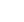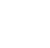# Linear Algebra I: Linear Equations

## Description

Systems of equations live at the heart of linear algebra. In this course you will explore fundamental concepts by exploring definitions and theorems that give a basis for this subject. At the start of this course we introduce systems of linear equations and a systematic method for solving them. This algorithm will be used for computations throughout the course as you investigate applications of linear algebra and more complex algorithms for analyzing them. Later in this course you will later see how a system of linear equations can be represented in other ways, which can reduce problems involving linear combinations of vectors to approaches that involve systems of linear equations. Towards the end of the course we explore linear independence and linear transformations. They have an essential role throughout our course and in applications of linear algebra to many areas of industry, science, and engineering. __

## Specific details

Category of Education Physical Science

## University

Georgia Institute of Technology

There are no comments posted here yetinfo@educom.net
 About Terms and ConditionsPrivacy PolicyRankingsAccreditations